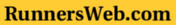Runner's Web JavaScript Race Time Predictor Calculator
This JavaScript race time predictor calculator allows you to predict your race time for a second distance by entering a time for a given distance and the second distance for which you wish a predicted time.

The JavaScript is based on the formula T2 = T1 x (D2/D1)1.07 where D1 is the race distance for which you have the time T1 and D2 is the second race distance. T2 is the time that the JavaScript will compute.